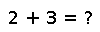# trunc

``````..  trunc([T,] x, [digits, [base]])

``trunc(x)`` returns the nearest integral value of the same type as ``x`` whose absolute
value is less than or equal to ``x``.

``trunc(T, x)`` converts the result to type ``T``, throwing an
``InexactError`` if the value is not representable.

``digits`` and ``base`` work as for :func:`round`.``````

## Examples

Checking you are not a robot: